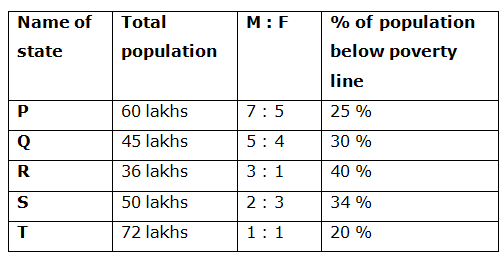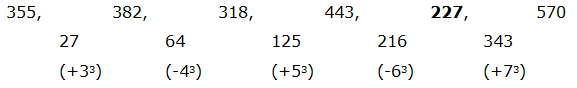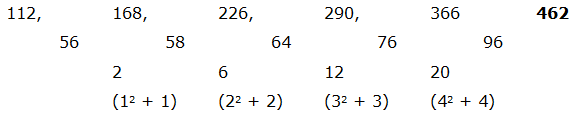# CWC/FCI Prelims 2019 – Quantitative Aptitude Questions (Day-04)

Dear Readers, Exam Race for the Year 2019 has already started, To enrich your preparation here we are providing new series of Practice Questions on Quantitative Aptitude – Section for CWC/FCI Exam. Aspirants, practice these questions on a regular basis to improve your score in aptitude section. Start your effective preparation from the right beginning to get success in upcoming CWC/FCI Exam.

[WpProQuiz 5421]

Directions (Q. 1 – 5): What value should come in place of question mark (?) in the following number series?

1) 355, 382, 318, 443, ? , 570

a) 278

b) 312

c) 356

d) 227

e) 334

2) 56, 28, 42, 105, ? , 1653.75

a) 367.5

b) 255.5

c) 412.25

d) 433.75

e) 289.25

3) 12, 25, 84, 361, ? , 11205

a) 1568

b) 1672

c) 1854

d) 1744

e) 2056

4) 150, 934, 258, 834, 350, ?

a) 734

b) 750

c) 776

d) 712

e) 728

5) 112, 168, 226, 290, 366, ?

a) 546

b) 438

c) 570

d) 462

e) 524

Directions (Q. 6 – 10): Study the following information carefully and answer the given questions:

The following table shows the total population in 5 different states, the ratio of male and female among them and the percentage of population below poverty line.6) Find the difference between the total male population in state P and R together to that of total female population in state Q and T together?

a) 15 lakhs

b) 22 lakhs

c) 6 lakhs

d) 10 lakhs

e) None of these

7) Find the ratio between the above poverty line population in state R to that of the below poverty line population in state S?

a) 108: 85

b) 75: 62

c) 52: 43

d) 11: 5

e) None of these

8) Find the total number of female population in state P, R and S together

a) 72 lakhs

b) 90 lakhs

c) 56 lakhs

d) 64 lakhs

e) None of these

9) The above poverty line population in state P and Q together is approximately what percentage of total population in state S?

a) 132 %

b) 153 %

c) 120 %

d) 105 %

e) 94 %

10) The below poverty line population in state R and T together is what percentage more/less than the total male population in state Q and S together?

a) 36 % more

b) 15 % less

c) 36 % less

d) 4 % more

e) 15 % more

Direction (1-5) :The difference is, +33, -43, +53, -63, +73,…

The pattern is, *1/2, *3/2, *5/2, *7/2, *9/2,…

The pattern is, *2 + 12, *3 + 32, *4 + 52, *5 + 72, *6 + 92,…

The pattern is, +282, -262, +242, -222, +202,…The difference of difference is, 12 + 1, 22 + 2, 32 + 3, 42 + 4,…

(or)

The difference of difference is, (1*2), (2*3), (3*4), (4*5)

Direction (6-10) :

The total male population in state P and R together

= > 60*(7/12) + 36*(3/4)

= > 35 + 27 = 62 lakhs

The total female population in state Q and T together

= > 45*(4/9) + 72*(1/2)

= > 20 + 36 = 56 lakhs

Required difference = 62 – 56 = 6 lakhs

The above poverty line population in state R

= > 36*(60/100)

The below poverty line population in state S

= > 50*(34/100)

Required ratio = [36*(60/100)]: [50*(34/100)]

= > 108: 85

The total number of female population in state P, R and S together

= > 60*(5/12) + 36*(1/4) + 50*(3/5)

= > 25 + 9 + 30 = 64 lakhs

The above poverty line population in state P and Q together

= > 60*(75/100) + 45*(70/100)

= > 45 + 31.5 = 76.5 lakhs

Total population in state S = 50 lakhs

Required % = (76.5/50)*100 = 153 %

The below poverty line population in state R and T together

= > 36*(40/100) + 72*(20/100)

= > 14.4 + 14.4 = 28.8 lakhs

The total male population in state Q and S together

= > 45*(5/9) + 50*(2/5)

= > 25 + 20 = 45 lakhs

Required % = [(45 – 28.8)/45]*100 = 36 % less